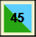## Sieve of Eratosthenes### Question I

Note that, given a natural number greater than $$1$$, the set of its divisors which are different from $$1$$ has at least one member (at least the number itself).

Let us then consider the following question:

1. Given a natural number greater than $$1$$, what is its least divisor (different from $$1$$)?

In what follows, whenever we speak of a least divisor we always mean a divisor different from $$1$$.

Let us think on some concrete examples. What is the smallest divisor of 12? And of 21? And of 35?

The following interactive application may help you answer the above questions. Try to reach a more general conclusion... By clicking on "View multiples of $$2$$" (respectively, $$3,5,7$$), the multiples of $$2$$ ($$3, 5, 7$$) greater than $$2$$ ($$3, 5, 7$$, respectively) are identified with a color. It is possible to select at the same time more than one option. For example, if the options "View multiples of $$2$$" and "View multiples of $$3$$" are selected, the numbers that are multiples of $$2$$ and $$3$$ are marked with two colors, and those that are multiples of only $$2$$ or only of $$3$$ will be marked only with one color (the color of the multiples of $$2$$ or of $$3$$ respectively).

The applet helps you answering all the questions above. Simply select the 4 options simultaneously (See multiples of $$2$$, $$3$$, $$5$$ and $$7$$. Then see what colors each number is couloured with and identify the color that corresponds to the smallest number. For instance, to find what is the smallest divisor of 30, after looking to the number 30 in, we see that 30 is a multiple of 2, 3, and 5 (because it is colored pink, blue, and green); thus its smallest divisor is $$2$$. On the other hand, the number $$45$$is colored blue and green, so it is a multiple of $$3$$ and $$5$$ and its smallest divisor is $$3$$.

In case you have not done it yet, compute also the smallest divisor of 12, 21 and 35. You might also do the same for other numbers.

Now suppose we had not that applet at our disposal. How could we find the least divisors?

$$12$$ is an even number, thus it is divisible by $$2$$ and this is clearly its smallest divisor.
We can of course conclude that the smallest divisor of any even number is $$2$$.

As for $$21$$, it is not divisible by $$2$$ (since it is odd) and it is divisible by $$3$$, since $$3 \times 7=21$$. Therefore $$3$$ is its smallest divisor.

Finally, $$35$$ is neither divisible by $$2$$ nor by $$3$$, since $$35:3=11$$ (remainder $$2$$). Further, since $$35$$ is not divisible by $$2$$, it is also not divisible by $$4$$. But it is divisible by $$5$$ (since $$5 \times 7=35$$), thus $$5$$ is the smallest divisor of $$35$$.